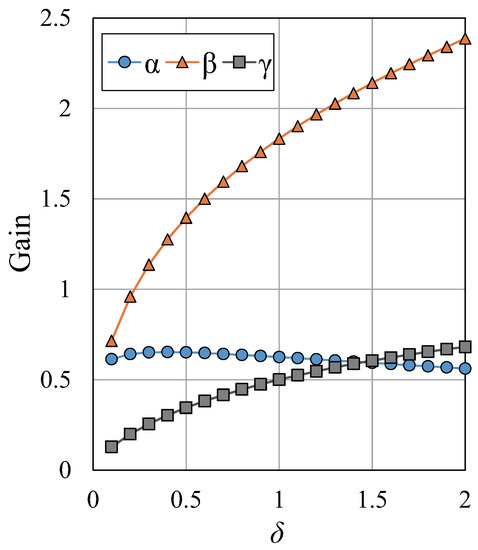Next Article in Journal
Influence of Contextual Factors on Soundscape in Urban Open Spaces
Previous Article in Journal
A Deeper Insight on the Stability of Water-Induced Reconstruction of Anatase (001) Surface
Open AccessArticle

# Correct Stability Condition and Fundamental Performance Analysis of the α-β-γ-δ Filter

byTakanori Shibata andKenshi Saho *Department of Intelligent Systems Design Engineering, Graduate School of Engineering, Toyama Prefectural University, Imizu, Toyama 939-0398, Japan
*
Author to whom correspondence should be addressed.
Appl. Sci. 2018, 8(12), 2523; https://doi.org/10.3390/app8122523
Received: 26 October 2018 / Revised: 29 November 2018 / Accepted: 5 December 2018 / Published: 6 December 2018
This paper theoretically analyzes the fundamental performance of a fourth-order steady-state moving object tracking filter, called an $α$ - $β$ - $γ$ - $δ$ filter. The $α$ - $β$ - $γ$ - $δ$ filter considers estimations of jerk (time-derivative of acceleration) in the motion of targets. First, regarding the stability conditions of the $α$ - $β$ - $γ$ - $δ$ filter, we prove that there are unstable cases even when the conventionally derived stability conditions are satisfied, and then we derive the correct stability conditions. Next, we analytically derive performance indices that indicate the steady-state errors for targets with typical motions. Based on the derived indices, the optimal performance of the $α$ - $β$ - $γ$ - $δ$ filter is theoretically analyzed and compared with that of the traditional second- and third-order steady-state tracking filters, i.e., $α$ - $β$ and $α$ - $β$ - $γ$ filters. Numerical analyses and simulations are used to verify the advantages and disadvantages of the $α$ - $β$ - $γ$ - $δ$ filter over the above-mentioned filters. The practicality of the use of jerk for the tracking filtering problem is revealed in this paper. View Full-Text
Show FiguresFigure 1

MDPI and ACS Style

Shibata, T.; Saho, K. Correct Stability Condition and Fundamental Performance Analysis of the α-β-γ-δ Filter. Appl. Sci. 2018, 8, 2523.